# NCERT Solutions for Class 8 Maths Chapter 13 Direct and Inverse Proportions

NCERT Solutions for Class 8 Maths Chapter 13 Direct and Inverse Proportions: You must have come across many situations where changes in one quantity result in changes in other quantities. In mathematics, two quantities are said to be in proportional if the values of two quantities are related in such a way that changes in one results in a corresponding change in another quantity. You must have observed that when you increase the speed of the car it takes lesser time to cover the same distance. So speed is inversely proportional to time with a constant distance. In NCERT solutions for class 8 maths chapter 13 direct and inverse proportions, you are going to deal with problems based on direct and indirect proportions. In this chapter, there are 2 exercises with 21 questions. The first exercise of this chapter cover problems based on the direct proportions and the second exercise cover problems based on the inverse proportions. All these questions are prepared in solutions of NCERT for class 8 maths chapter 13 direct and inverse proportions in a step-by-step manner. It will be very easy for you to understand the concept. There are solved examples, exercises, and daily life activities in the textbook for better understanding of this chapter. In CBSE NCERT solutions for class 8 maths chapter 13 direct and inverse proportions, you will deal with problems related to properties and applications of direct proportion and inverse proportion. You can find NCERT solutions from class 6 to 12 of science and maths by clicking on the above link.

## What is a Direct Proportion?

Two quantities 'a' and 'b' are said to be in direct proportion if the increase (decrease) in 'a' results in the increase (decrease) in 'b' in such a manner that the ratio of their corresponding values remains constant. That is if a/b= k

Example-  Sunil goes to the stationery shop to buy two pencils. The two pencils cost him Rs. 4. Next week again Sunil goes to the stationery shop to buy 6 pencils. The six pencils cost in Rs. 12. So, in this situation when the number of pencils increases causes gradual increases in the total cost. It means the number of pencils and total cost are directly proportional.What is Inverse Proportional?

Two values are Inversely proportional it means decreases in one value results in a gradual increase in other value. Example- If the distance is fixed, speed and time are inversely proportional to each other.

( If Distance is fixed)

## Topics of NCERT Class 8 Maths Chapter 13 Direct and Inverse Proportions-

• 13.1 Introduction
• 13.2 Direct Proportion
• 13.3 Inverse Proportion

## Solutions of NCERT for class 8 maths chapter 13 direct and inverse proportions topic 13.2 direct proportion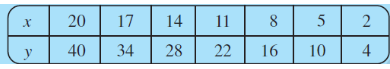If we want to know that they are directly proportional, then we calculate     :

.

Hence we can say that x and y are directly proportional as  cames out to be a constant equals .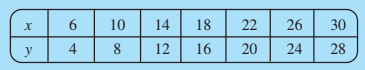If x and y are directly proportional then   must be equal to a constant value let say some  'k'.

Hence we then calculating:

,

as you can see that all these values are not equal hence,

we can say that x and y are not directly proportional.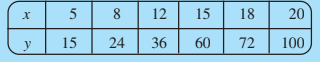Calculating   we get:

So, clearly  equals to   hence it is not equal to some constant value 'k'.

We can say that x and y are not directly proportional.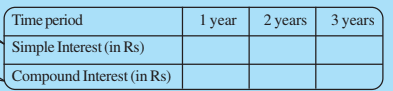Given that Principal (P) = 1000 and Rate (r) = 8% per annum(per year).

Calculating the Simple Interest:

The formula for the simple interest is =

So, for 1 year:

.

for 2 years:

.

similarly for 3 years:

.

Calculating the Compound Interest :

The formula for the compound interest is

.

So for 1 year:

.

for 2 years:

.

similarly for 3 years:

.

Hence we have

 Time period 1 year 2 year 3 year Simple Interest(in rupees) 80 160 240 Compound Interest(in rupees) 80 166.4 259.71

In case of simple interest

Simple interest is directly proportional with time.

While in case of compound interest:

does not give the same constant.

Compound interest is not directly proportional with time.

## CBSE NCERT solutions for class 8 maths chapter 13 direct and inverse proportions-Exercise: 13.1

We say that x and y are in direct proportion, if   or

Hence in the given problem we have,

Charges for the parking :

 y x 4 hours Rs. 60 8 hours Rs. 100 12 hours Rs. 140 24 hours Rs. 180

considering x to be charged to be paid and to be the parking time.

Then we calculate  ,

For:-    4 hours:                       8 hours :

12 hours :                  24 hours:

Clearly  is not equal to some constant 'k',  then we can say that

Parking charges are not in direct proportion to parking time.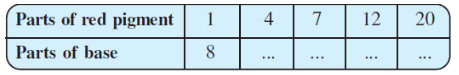Suppose the parts of red pigment is x and parts of base, is 'y'.

As the requirement of the number of parts of base for 1 part of red pigment is 8,

and as the parts of red pigment is increases, parts of base also increases in the same ratio. It is a case of direct proportion.

we can assume other parts of the base that will be required for red pigments as y1, y2, y3 and y4 for parts of pigment 4, 7, 12 and 20 respectively.We make use of the relation of type

That gives for the (i) case   or   .

32 parts of the base will be required for the 4 parts of the red pigment.

(ii) If parts of red pigment used is 7 then parts of base used will be

that gives  or    .

56 parts of the base will be required for the 7 parts of the red pigment.

(iii) for 12 parts of red pigment :

we have     or   .

so, 96 parts of the base will be required for the 12 parts of the red pigment.

(iv) for 20 parts of red pigment:

we have     or  .

Hence for the following parts of red pigment, parts of base are given :

 Parts of red pigment 1 4 7 12 20 Parts of base 8 32 56 96 160

As we know that mL of red pigment is directly proportional to the mL of base.

We have to calculate for the mL of red pigment used when we mix 1800mL of base,

So, for 1 part of red pigment requires 75mL of base, then we

.

So for 1800mL of the base, mL of the red pigment should be mixed

or

Hence for the 1800mL of base, we will mix 24 parts of red pigment.

Given that a machine is capable of filling 840 bottles in 6 hours. So, we can say that it is directly proportional to the time.

we can assume that bottles it will fill in five hours would be 'x'

So we got the relation;  ,

solving for x we will get        or   .

Machine will fill 700 bottles in 5 hours.We can calculate it easily,

Given that for 50,000 times enlarged, the photograph of a bacteria attains a length of 5cm.

So, we have to calculate the actual length of the bacteria(assume it to be 'x'cm) that is when the photograph is enlarged to 1 time

Knowing that the microscope's zoom has a direct relation with the length of bacteria observed So, we get the relation ;

Solving the equation for x we get;

The actual length of the bacteria is  which is so small to be observed through naked eyes.

Now, calculating the length of bacteria when it the photograph is enlarged 20,000 times,

assume it as 'y'.

So, we get this relation

solving for y we get;

.

So, if the photograph is enlarged 20,000 times only then the enlarged length would be 2cm.Given that the mast of the model ship is 9 m high, while the mast of the actual ship is 12 m.

If the length of the ship given is 28m then,

Length of the model ship can be obtained from the direct proportion relation of mast height and length of ship.

We obtained the relation:

So, by the relation we have;

Let us assume that the length of model ship is 'x

after solving this relation we get,

,

Length of the model ship is 21cm.

Here given that 2 kg of sugar contains  crystals.

So, we have to find the number of crystals in 5 kg of sugar as well as in 1.2 kg of sugar:

As here we will assume that all crystals have the same dimensions i.e., length, breadth, and width. Then the weight of sugar follows the direct proportion with the number of crystals as increasing the number of crystals there will be an increase in the weight also.

(i) For 5 kg sugar:

let the number of crystals be 'x' then,

we have the relation:

, calculating x from this relation,

.

Therefore 5 kg of sugar contains  sugar crystals.

(ii) For 1.2 kg sugar:

Let the number of cystals be 'y' then,

we have the relation:

. calculating similarly for y we get

.

Therefore 1.2 kg of sugar contains  sugar crystals.

Given that Rashmi has a road map with a scale of 1cm representing 18km.

So we have a direct relation of scale distance and distance driven in the road.

For 72 km she drove on a road, Distance covered in the map would be 'x' cm

,

.

Hence, For 72km driven in the road, we have to move a distance of 4cm on the map.

(i) the length of the shadow cast by another pole 10 m 50 cm high

(ii) the height of a pole which casts a shadow 5m long.

Consider there is a direct proportion relation of pole height with pole shadow.

So we have  5m 60cm high verticle pole that casts a shadow of 3m 20cm long .

hence,

(i) for the length of the shadow cast by another pole of 10 m 50cm high would be 'x' cm;

finding x from the equation we get;

.

Therefore for a 10m 50cm pole we would get a shadow of 600cm or 6m length.

(ii) The height of a pole which casts a shadow of 5m long let it be 'y'

similar relation holds here also, so we can apply it once more

,

we get  .

Thus, the height of a pole which casts a shadow of 5m long is 8m 75cm.

If the speed of the truck remains the same, then we can say that the distance travelled by the truck is directly proportional to the time.

After taking 5 hours the truck will travel a distance of let say 'x';

Hence we obtain the relation:

;

thus the truck would travel a distance of 168km in 5 hours.

NCERT solutions for class 8 maths chapter 13 direct and inverse proportions topic 13.3 inverse proportion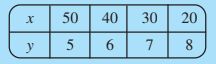Finding if x and y are in inverse proportion:

Two quantities x and y are said to vary in inverse proportion, if there exists a relation of the type xy = k between them, k being a constant

so, calculating xy for each case,

we have

,

clearly xy is not equal to a constant value 'k',

hence x and y are not inversely proportional.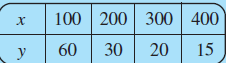Finding if x and y are in inverse proportion:

Two quantities x and y are said to vary in inverse proportion, if there exists a relation of the type xy = k between them, k being a constant

so, calculating xy for each case,

we have

,

clearly xy is equal to a constant value 'k=6000',

hence x and y are inversely proportional.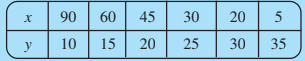Finding if x and y are in inverse proportion:

Two quantities x and y are said to vary in inverse proportion, if there exists a relation of the type xy = k between them, k being a constant

so, calculating xy for each case,

we have

clearly xy is not equal to a constant value 'k',

hence x and y are not inversely proportional.

## NCERT solutions for class 8 maths chapter 13 direct and inverse proportions-Exercise: 13.2

Question:1 Which of the following are in inverse proportion?

(i) The number of workers on a job and the time to complete the job.

## (ii) The time taken for a journey and the distance travelled in a uniform speed.

(iii) Area of cultivated land and the crop harvested.

(iv) The time taken for a fixed journey and the speed of the vehicle.

(v) The population of a country and the area of land per person.

(i) As the number of workers on a job increases the time taken to complete the job decreases, hence it is an inverse proportion.

(ii) Distance and time are directly proportional to each other as time increases you could travel more distance compared to if you get less time to travel. Hence it is not an inverse proportion.

(iii) Both area of cultivated land and crop arvested are directly proportional, more the area cultivated more crop harvest. Hence it is not an inverse proportion.

(iv) With more speed if you are travelling lesser the time taken for a fixed journey to complete. Hence it is an inverse proportion.

(v) The population of a country if increases then there would be lesser area available per person, Hence it is an inverse proportion.

 Number of winners 1 2 4 5 8 10 20 Prize for each winner (in Rs.) 1,00,000 50,000 .... .... .... .... ....

Let us assume that for the number of winners 4, 5, 8, 10 and 20 are x1, x2, x3, x4, and x5 respectively and given that the prize money of Rs. 1,00,000 is to be divided equally amongst the winners.

Thus we have,

For 4 number of winners:

So Rs. 25,000 to be distributed among each.

For 5 number of winners:

So Rs. 20,000to be distributed among each.

For 8 number of winners:

So Rs. 12,500 to be distributed among each.

For 10 number of winners:

So Rs. 10,000 each would get.

similarly for 20 number of winners:

So Rs. 5000 each would get.

Hence we have;

 Number of winners (x) 1 2 4 5 8 10 20 Prize for each winner (in ? ) (y) 1,00,000 50,000 25,000 20,000 12,500 10,000 5,000

Two quantities x and y are said to be in inverse proportional if they satisfy the given relation;

xy=k; where k is a constant.

Calculating xy:

Clearly, we can see that the prize money given to an individual winner is inversely proportional.

Are the number of spokes and the angles formed between the pairs of consecutive spokes in inverse proportion?Number of spokes 4 6 8 10 12 Angle between a pair of consecutive .... .... ....

(i) Calculating the angle formed when using a different number of spokes:

The angle formed when using 8, 10, and 12 number of spokes a1,a2, and a3 respectively.

Hence, we have

For 8 number of spokes:

For 10 number of spokes:

For 12 number of spokes:

 Number of spokes (x) 4 6 8 10 12 Angle between a pair of consecutive spokes (y) 90° 60° 45° 36° 30°

Calculating xy:

Hence we say that the number of spokes (x) and the angle formed (y) between them are inverse proportion to each other.

Calculate the angle between a pair of consecutive spokes on a wheel with 15 spokes.Number of spokes 4 6 8 10 12 Angle between a pair of consecutive .... .... ....

The angle between a pair of consecutive spokes on a wheel with 15 spokes is calculated as:

as we know the constant value  then we can easily calculate the angle for 15 spokes:

Hence angle made is 24 degrees.

How many spokes would be needed, if the angle between a pair of consecutive spokes is ?Number of spokes 4 6 8 10 12 Angle between a pair of consecutive .... .... ....

If the angle between a pair of consecutive spokes is   then,

Number of spokes needed would be

Hence the required number of spokes for having 40 degrees of angle is 9

Given that the box of sweets is divided among 24 children, getting 5 sweets each.

If the number of children is reduced by 4 then the number of children now is .

As here if the number of children increases then the number of sweets they will get decreases hence we can say that there exists an inverse relationship between them.

Hence,

if we assume:

Number of children before (x1)  = 24

Number of sweets each would get before (y1) = 5

and number of children after reduction (x2) = 20

and the number of sweets each would get after reduction is y2

Then the relation holds;

or    or   .

Hence each child will get 6 number of sweets.

The farmer has 20 animals to feed for 6 days and after that farmer added 10 more animals to feed that counts to (20+10 = 30). So, assume that food will now long last to 'x' days.

As the number of animals increases the required food increases but the day up to which the food will long last decreases.

Hence there exist an inverse proportion between the number of days and the number of animals.

So, we can write the relation as:

or     days.

The food will last for about 4 days if 30 animals are there.

Here the situation is given that a contractor estimates that 3 persons could rewire Jasminder's house in 4 days.

Now, as the number of person increases the time they take to complete the job will decrease.

Hence there is an inverse relationship among the number of persons and the time they took.

Jasminer now uses 4 persons instead of 3, then we can assume the time 4 persons will take be 'x'

So, we can write the relation as:

. or     .

Thus, the 4 persons complete the job in 3 days.We can easily calculate the required number of boxes to be filled, let us assume it to be 'x'

Given that a batch of bottles were packed in 25 boxes with 12 bottles in each box,                    Hence the total number of bottles will be .

So, as they produced the same number of bottles every batch and as the number of boxes increases, the bottles in each box decrease.

Hence there holds an inverse relation here,

or             boxes.

If the batch is packed using 20 bottles in each box then 15 boxes would be filled.

Given that a factory requires 42 machines to produce a given number of articles in 63 days.

Then we know that as the number of machines increases the time taken to produce a given number of articles decreases. Hence there holds an inverse relation here,

And let the number of machines required to produce the same number of articles in 54 days be 'x'.

Then the relation;

or         .

Hence 49 machines would be required to produce the articles in 54 days.

Given that a car has a speed of 60 km/h which is travelling to a destination and takes 2 hours to complete it.

Speed and time are inversely related to each other.

Assume the time it would take when travelling at 80km/h be 'x'

Therefore we can write the equation when the car travels at the speed of 80 km/h as:

or      or

Question:10(i) Two persons could fit new windows in a house in 3 days.

One of the persons fell ill before the work started. How long would the job take now?

Here, given that 2 persons could fit new windows in a house in 3 days.

(i) 1 person has fallen ill so, now the number of persons remaining is only one. Assume that the only person which is working takes the time of 'x' days.

hence we could write the inverse relation as;

or       .

One person will take 6 days to complete that window job.

Question:10(ii) Two persons could fit new windows in a house in 3 days.

How many persons would be needed to fit the windows in one day?

(ii) So, now we are calculating the number of persons that would be needed to fit the windows in one day. Let it be 'y'.

So from previous part (i) we have the relation;  .

or    .

hence the required number of persons would be 6.

Question:11. A school has periods a day each of minutes duration. How long would each period be, if the school has  periods a day, assuming the number of school hours to be the same?

Given :

A school has 8 periods a day each of 45 minutes duration.

So, if the school hours of school is fixed then there exists an inverse relationship between each period duration and the number of periods.

We can take the time of each period to be 't' if the school has 9 periods a day.

Hence we can write the relation as;

or      .

The time of each period would be 40 minutes when 9 periods are there in one day.

## NCERT solutions for class 8 maths: Chapter-wise

 Chapter -1 NCERT solutions for class 8 maths chapter 1 Rational Numbers Chapter -2 Solutions of NCERT for class 8 maths chapter 2 Linear Equations in One Variable Chapter-3 CBSE NCERT solutions for class 8 maths chapter 3 Understanding Quadrilaterals Chapter-4 NCERT solutions for class 8 maths chapter 4 Practical Geometry Chapter-5 Solutions of NCERT for class 8 maths chapter 5 Data Handling Chapter-6 CBSE NCERT solutions for class 8 maths chapter 6 Squares and Square Roots Chapter-7 NCERT solutions for class 8 maths chapter 7 Cubes and Cube Roots Chapter-8 Solutions of NCERT for class 8 maths chapter 8 Comparing Quantities Chapter-9 NCERT solutions for class 8 maths chapter 9 Algebraic Expressions and Identities Chapter-10 CBSE NCERT solutions for class 8 maths chapter 10 Visualizing Solid Shapes Chapter-11 NCERT solutions for class 8 maths chapter 11 Mensuration Chapter-12 Solutions of NCERT for class 8 maths chapter 12 Exponents and Powers Chapter-13 CBSE NCERT solutions for class 8 maths chapter 13 Direct and Inverse Proportions Chapter-14 NCERT solutions for class 8 maths chapter 14 Factorization Chapter-15 Solutions of NCERT for class 8 maths chapter 15 Introduction to Graphs Chapter-16 CBSE NCERT solutions for class 8 maths chapter 16 Playing with Numbers

## Benefits of NCERT solutions for class 8 maths chapter 13 direct and inverse proportions-

• You will also know different ways to solve the problems.

• You will also get solutions to the practice questions given below every topic which will give you conceptual clarity.

• You will also get some short tricks and tips to solve some specific problems.

• In one of the questions of CBSE NCERT solutions for class 8 maths chapter 13 direct and inverse proportions, you will learn to calculate simple and compound interest which may be useful in real life too.

Happy learning!!!